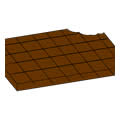#### You may also like### Diophantine N-tuples

Can you explain why a sequence of operations always gives you perfect squares?### DOTS Division

Take any pair of two digit numbers x=ab and y=cd where, without loss of generality, ab > cd . Form two 4 digit numbers r=abcd and s=cdab and calculate: {r^2 - s^2} /{x^2 - y^2}.### Sixational

The nth term of a sequence is given by the formula n^3 + 11n . Find the first four terms of the sequence given by this formula and the first term of the sequence which is bigger than one million. Prove that all terms of the sequence are divisible by 6.

# Chocolate 2010

##### Age 14 to 16Challenge LevelFirst of all, pick the number of times a week that you would like to eat chocolate (try for more than once but less than 10 times as more than this is simply greedy).
Multiply this number by 2 (just to be bold).